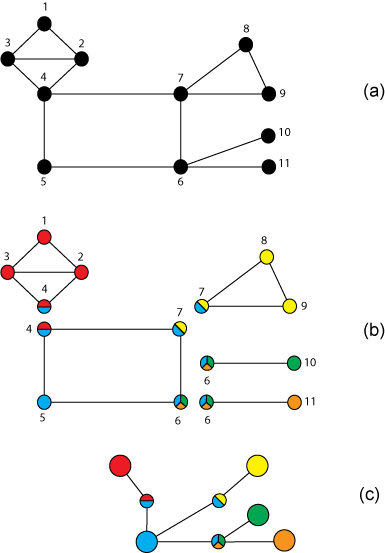# biconncomp

## 语法

``bins = biconncomp(G)``
``bins = biconncomp(G,'OutputForm',form)``
``[bins,iC] = biconncomp(___)``

## 说明

``bins = biconncomp(G)` 以 bin 形式返回图 `G` 的双连通分量。bin 编号指示图中的每个边所属的双连通分量。`G` 中的每条边属于一个双连通分量，而 `G` 中的节点可以属于多个双连通分量。如果两个节点无论从图中删除哪一个都不会使它们断开连接，则说明它们属于同一个双连通分量。`

``bins = biconncomp(G,'OutputForm',form)`（其中 `form` 为 `'cell'`）以元胞数组形式返回输出，因此 `bins{j}` 包含分量 `j` 中所有节点的节点 ID。`form` 的默认值为 `'vector'`。`

``[bins,iC] = biconncomp(___)` 还返回节点索引 `iC`，指示哪些节点是割点（也称为关节点）。`

## 示例

```s = [1 1 2 2 3 4 4 5 6 6 7 7 8]; t = [2 3 3 4 4 5 7 6 7 10 8 9 9]; G = graph(s,t); p = plot(G,'LineWidth',2);```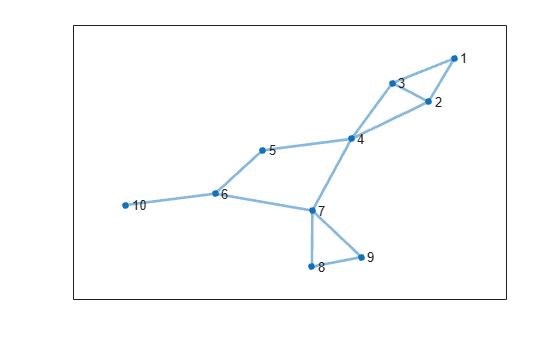`p.EdgeCData = biconncomp(G);`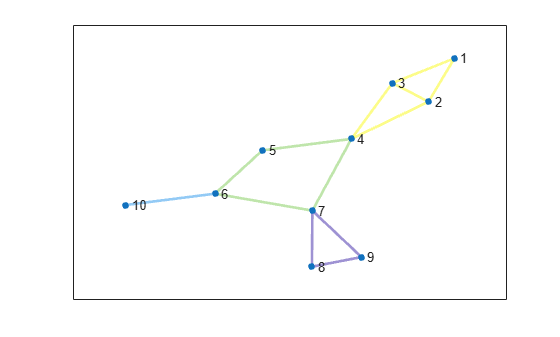```s = [1 1 2 2 3 4 4 5 6 6 7 7 8]; t = [2 3 3 4 4 5 7 6 7 10 8 9 9]; G = graph(s,t); plot(G)```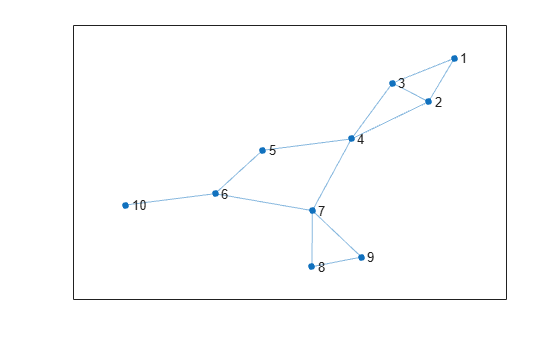```bincell = biconncomp(G, 'OutputForm', 'cell'); n = length(bincell); for ii = 1:n subplot(2,2,ii) plot(subgraph(G, bincell{ii}), 'NodeLabel', bincell{ii}); end```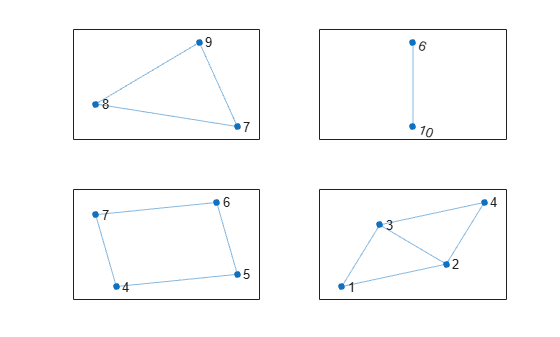```s = [1 1 2 2 3 4 4 5 6 6 7 7 8]; t = [2 3 3 4 4 5 7 6 7 10 8 9 9]; G = graph(s,t); p = plot(G);````[edgebins,iC] = biconncomp(G)`
```edgebins = 1×13 4 4 4 4 4 3 3 3 3 2 1 1 1 ```
```iC = 1×3 4 6 7 ```

`highlight(p, iC)`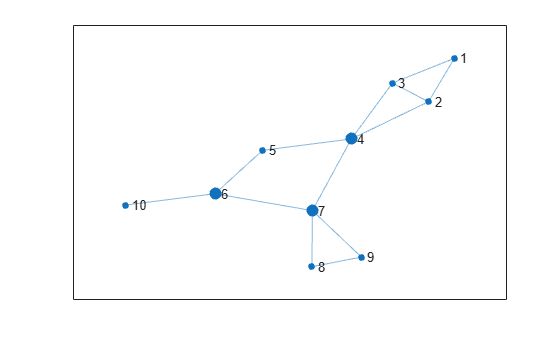## 输入参数

`'vector'`（默认值）`bins` 是数值向量，指示每条边所属的双连通分量。
`'cell'``bins` 是元胞数组，`bins{j}` 包含属于分量 `j` 的所有节点的节点 ID。

## 输出参数

• 如果 `OutputForm``'vector'`（默认值），则 `bins` 是数值向量，指示每个边所属的连通分量 (bin)。自环边分配给 bin `0`，因为这些边不属于任何双连通分量。

• 如果 `OutputForm``'cell'`，则 `bins` 是元胞数组，`bins{j}` 包含属于分量 `j` 的所有节点的节点 ID。

## 详细信息

### 双连通分量

• (a) 一个具有 11 个节点的无向图。

• (b) 图的五个双连通分量，原图的割点通过不同颜色表示各自所属的分量。

• (c) 图的块割点树，其中包含代表各个双连通分量的节点（用纯色大圆表示）和代表各个割点的节点（用多色小圆表示）。在块割点树中，每个割点与它所属的每个分量之间由一条边相连。